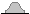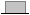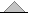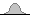# Post-Processing Results in POSTFEKO

After solving the Three_Spheres_VPol_MoM.cfx model, the post-processing is done in POSTFEKO using the PF_ISAR.lua macro.

The PF_ISAR.lua application macro uses the following input parameters:
Far field request
Select the RCS far field request to process.
Windowing function
•Hamming
•Dirichlet
•Bartlett
•Blackman
Interpolate to Cartesian grid
The 2D FFT requires that the X and Y distances should be uniformly spaced. The basic ISAR implementation assumes that the angle range is small enough such that the polar sampling approximates a Cartesian grid. The small angle range assumption can be relaxed by transforming the polar grid to Cartesian. This reduces smearing and shift of the scattering points and increasing accuracy of the resulting ISAR image.
Component
Specify which E-field component to use to generate the ISAR result. Supported options are:
• Theta
• Phi
• LHC (Left Hand Circular)
• RHC (Right Hand Circular)
Image offset
Offset distance of the ISAR image above the plane wave workplane.
Resample factor
Factor used to up sample the image resolution to create a smoother image with more points. Note it does not change the underlying image resolution based on the frequency and angular information.
Create 3D view
Generates a normal view of the partially transparent ISAR image with RCS data displayed in dB.
Find local maxima
Calculates the specified number of local maxima in descending order in the ISAR image.
Export local maxima to CSV
Export the specified number of local maxima to a CSV file. Find local maxima has to be checked for this to work.
Number of local maxima
The number of local maxima to find.
After accepting the input from the main form, the application macro will process the RCS data and display a dialog to select the viewing angle from the list. The default viewing angle is the average of the start angle and end angle.
Note: A different viewing angle could reduce the maximum angular data range.
With the viewing angle specified, the application macro will calculate the maximum angular range that can be used. The user can then select an angular range from the list.
Note: The angular range affects the cross-range resolution.

The ISAR images associated with the XY_plane and UV_plane solution configurations are shown below. For this example, a resampling factor of 2 was used with default values for the rest of the input parameters.

The ISAR dataset can also be used to generate 2D graphs, such as the image below showing the RCS versus X position corresponding to each sphere’s Y coordinate.